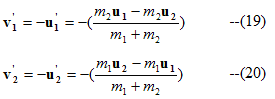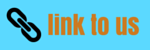# System of particles and Collision

## (11) Collisions

• Collision between two particles is defined as mutual interaction between particles for a short interval of time as a result of which energy and momentum of particle changes.
• Collision between two billiard balls or between two automobiles on road are few examples of collisions from our everyday life. Even gas atoms and molecules at room temperature keep on colliding against each other.
• For the collision to take place , physical contact is not necessary. In cas of Rutherford alpha scattering experiment , the alpha particles are scattered due to electrostatic interaction between the alpha particles and the nucleus from a distance i.e., no physical contact occurs between the alpha particles and the nucleus.
• Thus , in physics collision is said to have occured , if two particles physically collide with each other or even when the path of motion of one particle is affected by other.
• In the collision of two particles law of conservation of momentum always holds true but in some collisions Kinetic energy is not always conserved.
• Hence collisions are of two types on the basis of conservation of energy.
(i) Perfectly elastic collision
• Those collisions in which both momentum and kinetic energy of system are conserved are called elastic collisions for example elastic collision occurs between the molecules of a gas. This type of collision mostly takes place between the atoms, electrons and protons.
• Characterstics of elastic collision
(a) Total momentum is conserved.
(b) Total energy is conserved.
(c) Total kinetic energy is conserved.
(d) Total mechanical energy is not converted into any other form of energy.
(e) Forces involved during interaction are conservative in nature.
• Consider two particles whose masses are m1 and m2 respectively and they collide each other with velocity u1 and u2 and after collision their velocities become v1 and v2 respectively.
• If collision between these two particles is elastic one then from law of conservation of momentum we have
m1u1 + m2u2 = m1v1 + m2v2
and from the law of conservation of energy we have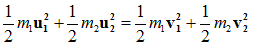(ii) Perfectly inelastic collision
• Those collisions in which momentum of system is conserved but kinetic energy of the system is not conserved are known as inelastic collision.
• Here in inelastic collision two bodies stick to each other after collision as a bullet hit its target and remain embedded in the target.
• In this case some of the kinetic energy is converted into heat or is used up in in doing work in deforming bodies for example when two cars collide their metal parts are bet out of shape.
• Characterstics of inelastic collision
(a) Total momentum is conserved.
(b) Total energy is conserved.
(c) Total kinetic energy is not conserved.
(d) A part or whole of whole mechanical energy may be converted into other forms of energy.
(e) Some or all forces involved during interaction are non-conservative in nature.
• Consider two particles whose masses are m1 and m2 respectively and they collide each other with velocity u1 and u2 respectively.
• If the collision between these two particles is inelastic then these two particles would stick to each other and after collision they move with velocity v then from law of conservation of momentum we have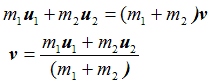• Kinetic energy of particles before collisions is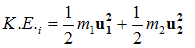and kinetic energy of particles after collisions is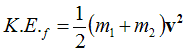by law of conserevation of energy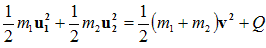where Q is the loss in kinetic energy of particles during collision.

## (12) Head on elastic collision of two particles in L-frame of refrance

• Consider two particles whose masses are m1 and m2 respectively and they collide each other with velocity u1 and u2 and after collision their velocities become v1 and v2 respectively.
• Collision between these two particles is head on elastic collision. From law of conservation of momentum we have
m1u1 + m2u2 = m1v1 + m2v2                      (1)
and from law of conservation of kinetic energy for elastic collision we have(2)
rearranging equation 1 and 2 we get
m1(u1-v1)= m2(v2-u2)                     (3)
and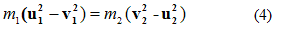dividing equation 4 by 3 we get
u1 + v1 = u2 + v2
u2 - u1 = -(v2 - v1)                     (5)
where (u2 - u1) is the relative velocity of second particle w.r.t. first particle before collision and (v2 - v1) is the relative velocity of second particle w.r.t. first after collision.
• From equation 5 we come to know taht in a perfectly elastic collision the magnitude of relative velocity remain unchanged but its direction is reversed. With the help of above equations we can find the values of v2 and v1 , so from equation 5
v1 = v2 - u1 + u2                     (6)
v2 = v1 + u1 - u2                     (7)
Now putting the value of v1 from equation 6 in equation 3 we get
m1(u1 - v2 + u1 - u2) = m2(v2 - u2)
On solving the above equation we get value of v2 as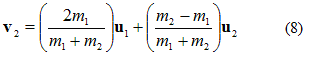• Similarly putting the value of v2 from equation 7 in equation 3 we get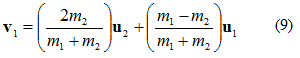• Total kinetic energy of particles before collision is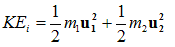and total K.E. of particles after collision is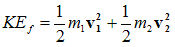• Ratio of initial and final K.E. is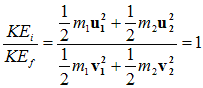• Special cases
Case I: When the mass of both the particles are equal i.e., m1 = m2 then from equation 8 and 9 , v2=u1 and v1=u2. Thus if two bodies of equal masses suffer head on elastic collision then the particles will exchange their velocities. Exchange of momentum between two particles suffering head on elastic collision is maximum when mass of both the particles is same.

Case II: when the target particle is at rest i,e u2=0
From equation (8) and (9)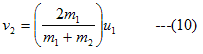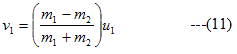Hence some part of the KE which is transformed into second particle would be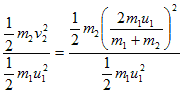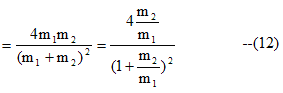when m1=m2,then in this condition v0=0 and v2=u1 and part of the KE transferred would be
=1

Therefore after collisom first particle moving with initial velocity u1 would come to rest and the second particle which was at rest would start moving with the velocity of first particle.Hence in this case when m1=m2 transfer of energy is 100%.if m1 > m2 or
m1 < m2 ,then energy transformation is not 100%

Case III:
if m2 >>>> m1 and u2=0 then from equation (10) and (11)
v1 ≅ -u1 and v2=0                     (13)
For example when a ball thrown upwards collide with earth

Case IV:
if m1 >>>> m2 and u2=0 then from equation (10) and (11)
v1 ≅ u1 and v2=2u1                     (14)

Therefore when a heavy particle collide with a very light particle at rest ,then the heavy particle keeps on moving with the same velocity and the light particle come in motion with a velocity double that of heavy particle

## (13) Head on collison of two particles in C frame of refrence

• Consider two particles of mass m1 and m2 having position vectors r1 and r2 respectively And position vector of the CEnter of mass of the system would be Rcm
then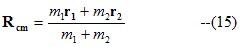Velocity of the center of mass would be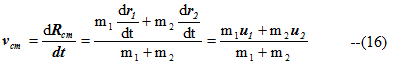Intial velocity of the m1 w.r.t center of mass frame of refrence is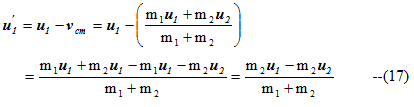Similarly Intial velocity of m2 w.r.t center of mass frame of refrence is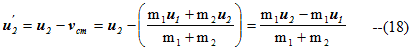• Total linear momentum before collison in absence of external force in C frame of refrence would be
=m1u1' +m2u2'
=0

So u2'=(m1/m2)u1'

• If v1' and v2' are the velocites of mass m1 and m2 respectively after collision then by law of conservation of linear momentum
m1v1' +m2v2'=0
v2'=(m1/m2)v1'

Since the collision is elastic,Kinetic energy will be conserved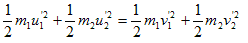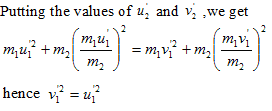From which |v1'|=|u1'| and |v2'|=|u2'|

hence after collison velocities of particles remain unchanged in center of mass frame of refrence.If the collision is one dimmension then because of the collsion direction of these would be opposite to that of their intial velocites